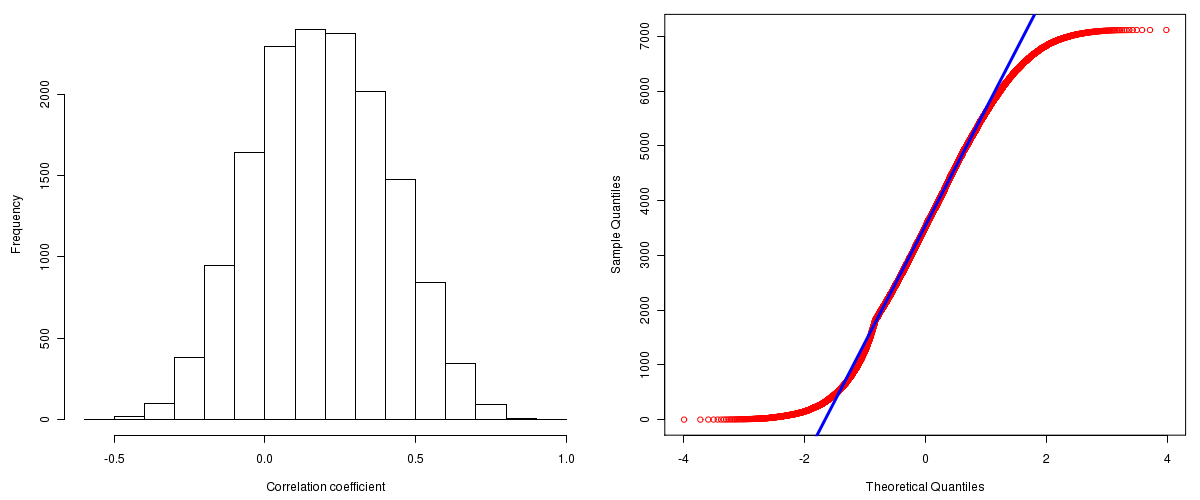Uterine Corpus Endometrioid Carcinoma: Correlations between copy number and mRNA expression
Maintained by John Zhang (Dana-Farber Cancer Institute)
Overview
Introduction

A TCGA sample is profiled to detect the copy number variations and expressions of genes. This pipeline attempts to correlate copy number and expression data of genes across samples to determine if the copy number variations also result in differential expressions. This report contains the calculated correlation coefficients based on measurements of genomic copy number (log2) values and intensity of the expressions of the corresponding feature across patients. High positive/low negative correlation coefficients indicate that genomic alterations result in differences in the expressions of mRNA the genomic regions transcribe.

Summary

The correlation coefficients in 10, 20, 30, 40, 50, 60, 70, 80, 90 percentiles are -0.09654, -0.00476, 0.06168, 0.1255, 0.186, 0.24686, 0.3128, 0.3866, 0.48244, respectively.

Results
Correlation results

Number of genes and samples used for the calculation are shown in Table 1. Figure 1 shows the distribution of calculated correlation coefficients and quantile-quantile plot of the calculated correlation coefficients against a normal distribution. Table 2 shows the top 20 features ordered by the value of correlation coefficients.

Table 1.  Counts of mRNA and number of samples in copy number and expression data sets and common to both

Category Copy number Expression Common
Sample 363 54 53
Genes 29390 17815 15551

Figure 1.  Summary figures. Left: histogram showing the distribution of the calculated correlations across samples for all Genes. Right: QQ plot of the calculated correlations across samples. The QQ plot is used to plot the quantiles of the calculated correlation coefficients against that derived from a normal distribution. Points deviating from the blue line indicate deviation from normality.Table 2.  Get Full Table Top 20 features (defined by the feature column) ranked by correlation coefficients

feature r p-value q-value chrom start end geneid
C19orf12 0.9176 0 0 19 34883910 34897971 83636
POP4 0.8786 0 0 19 34789041 34798547 10775
C17orf37 0.8646 0 0 17 35138935 35140314 84299
GSTT1 0.8524 4.44089209850063e-16 7.91356668707678e-13 22 22706141 22714231 2952
SAP30BP 0.828 2.04281036531029e-14 2.91219254084425e-11 17 71174994 71215734 29115
LCMT1 0.8046 3.90354415458205e-13 4.63735007862699e-10 16 25030548 25097052 51451
PSMB4 0.8025 4.9737991503207e-13 5.06468267972914e-10 1 149638665 149641036 5692
SCNM1 0.7925 1.54098955817972e-12 1.37300382020782e-09 1 149405141 149408238 79005
ASXL1 0.7901 2.00151006879423e-12 1.58517533594022e-09 20 30409821 30490783 171023
POLR2I 0.7859 3.13882253522024e-12 2.23732357377035e-09 19 41296451 41298046 5438
STARD3 0.784 3.86002341201674e-12 2.50126260523896e-09 17 35046938 35073263 10948
UBA52 0.781 5.27311527775964e-12 2.95626501993228e-09 19 18543668 18547050 7311
MAP2K4 0.7808 5.39168709678961e-12 2.95626501993228e-09 17 11864860 11987776 6416
RANBP10 0.7797 6.0382809863313e-12 3.06265582212121e-09 16 66314506 66398056 57610
ISG20L2 0.779 6.44506670255396e-12 3.06265582212121e-09 1 154959037 154964329 81875
ZNF687 0.778 7.18092252327551e-12 3.19905933325079e-09 1 149521415 149531005 57592
SUMO2 0.7757 9.07141028960723e-12 3.80353945216276e-09 17 70675420 70690693 6613
B4GALT3 0.7735 1.12305720278982e-11 4.44724862109966e-09 1 159407725 159413938 8703
BECN1 0.7705 1.51754164789963e-11 5.693103175385e-09 17 38215678 38229807 8678
C19orf2 0.7675 2.02473593446939e-11 7.21606491927783e-09 19 35125265 35198456 8725
Methods & Data
Input

Gene level (TCGA Level III) expression data and copy number data of the corresponding loci derived by using the CNTools package of Bioconductor were used for the calculations. Pearson correlation coefficients were calculated for each pair of genes shared by the two data sets across all the samples that were common.

Correlation across sample

Pairwise correlations between the log2 copy numbers and expressions of each gene across samples were calculated using Pearson correlation.